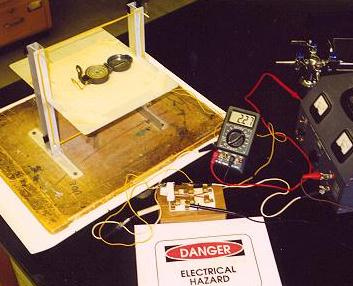Magnetic Field of Earth (Be)

Obj: Measure the horizontal component of Earth's Magnetic Field

Introduction: A magnetic compass needle will align itself with the horizontal component of the earth's magnetic field. If there are other magnetic fields around the compass, its alignment will represent the resultant of the several fields. This experiment will use the known field of a square coil and the resultant field to determine the earth's unknown magnetic field.

coil field + earth field = resultant field

Materials: compass, DC power supply, 1 Ohm (10 Watt) power resistor, coil setup as shown below, ammeter, reversing switchMethods

1. Place the compass in the center of the aluminum table coil as shown above.

2. Align the compass to the earth's N/S  poles and with the coil.

Number turns coil (n) = ____; length of wire on one side = ______ m

3. Energize and record the data in 0.5 amp increments up to approximately 4 amps, alternating the current flow, then finding the average compass deviation (q) from the earth's field.

Results and Analysis

1. Plot (nI) vs tanq. Calculate the slope.

2. Calculate Be using the equation for a toroidal solenoid coil:

Be= Bcoil / tanq

Let µo = 4 x10-7 Wb / Amp meter (permeability constant of free space).

[NOTE: (nI) / tanq is the slope of the line.]

3. Show that the units from your equation are Wb/m2 = tesla (T)

4. Calculate % error using up-to-date U.S. Geological Survey data from the internet or Geomagix (free download from Internet)

Back to the Brockport High School Science Department